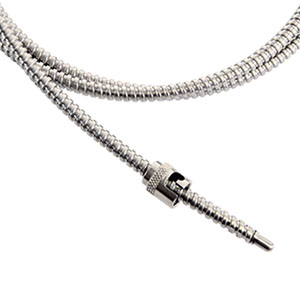Style S12With spring-loaded bayonet locking mechanism. Bayonet fitting is adjustable along its flexible armored cable, giving it unlimited adjustability and excellent flexibility.

• Two- or three-wire
• Resistance: 100Ω at 0°C
• Alpha curve: 0.00385Ω/Ω/°C
• Tolerance at 0°C: ±0.12% (±0.25°C)
• Range: -50 to 260°C (-58 to 500°F)
Back to Specialty

### Specifications

Specifications:  RTD

• Two- or three-wire
• Resistance: 100Ω at 0°C
• Alpha curve:  0.00385Ω/Ω/°C
• Tolerance at 0°C:  ±0.12% (±0.25°C)
• Range:  -50 to 260°C (-58 to 500°F)

Specifications:  Thermistor
• Metal oxide, sintered and encapsulated
• Negative temperature coefficient
• Non-linear temperature/resistance curve
• Resistance at 25°C (77°F) and ranges

Construction:
• 10 = 6 inch adjustable spring style
• 11 = 12 inch adjustable spring style
• 12 = Adjustable armor style
• 25 = Cartridge with flange
• 50 = Open air
• 55 = Open air with flange
• 80 = Surface mount
Diameter:
• D = 0.188
• A = Not applicable: surface mount
Element Type:
• Element Type
• C = RTD 2-wire No. 3850
• N = Thermistor No. 12
• D = RTD 3-wire No. 3850
• P = Thermistor No. 16
• M = Thermistor No. 11
• L4 = Fiberglass and SS armor
• M4 = Fiberglass
• N4 = Fiberglass and SS overbraid
• T2 = PFA or TFE
Termination:
A = Not applicable, C = 1.5 (required for VAT construction: No. 10, 11, 12), D = 2.0, L = 5.5, T = 9.0, E = 2.5, M = 6.0, U = 9.5, F = 3.0,N = 6.5, W = 10.0, G = 3.5, P = 7.0, Y = 11.0, H = 4.0, Q = 7.5, Z = 12.0, J = 4.5, R = 8.0, K = 5.0, S = 8.5